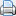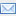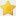# One Rep MaxHow much weight you can lift for one repetition is known as your one rep max, or 1RM . When you determine your one rep max, you can use a percentage of this to figure out how much weight to use for different exercises.

Many fitness training programs require the exerciser to lift some percentage of his/her one rep max. However, the risk of injury when attempting a 1RM may be higher than when performing multiple rep sets.

It's best for beginners to guess how much weight you need and adjust it throughout the workout. If your an intermediate or advanced weight lifter and are using this method there are various proposals for ways to calculate an approximation of the 1RM.

For example, one common and simple formula is:

((number of reps / 30) + 1) x weight used.

For example, if a person can lift 50 pounds on given exercise 15 times, the estimated one rep max would be ((15 / 30) + 1) * 50 = 75 pounds. If this person were to follow a fitness program using 80% of their one rep max in this exercise, they would then choose the weight 0.8 * 75 = 60 pounds.

This kind of calculation may not always produce accurate results, but can be used as starting point. You can then increase or decrease the weight as needed to perform the number of reps that you wish to follow in your training program.PrintEmailFavourites